## 16 January 2014

A triangle is a closed figure made of three line segments. Every triangle has three sides, three angles, and three vertices. These are known as the parts of a triangle. The sides and the angles of every triangle differ from one another; therefore, they do not look alike.
Triangles can be classified based on their sides and angles.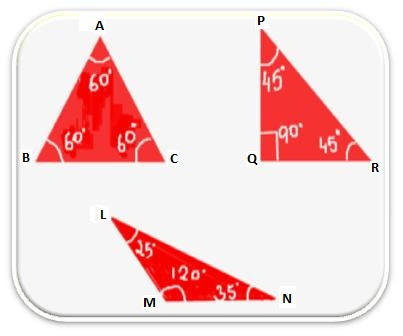• Based on their sides, there are equilateral,isosceles and scalene triangles.
• Based on their angles, there are acuteobtuse and right-angled triangles.
Equilateral triangle: A triangle in which all the sides are equal is called an equilateral triangle. All the three angles of an equilateral triangle are also equal, and each measures 60°.
Isosceles triangle: A triangle in which any two sides are equal is called an isosceles triangle. In an isosceles triangle, the angles opposite the equal sides are called the base angles, and they are equal.
Scalene triangle: A triangle in which no two sides are equal is called an Scalene triangle.
Acute-angled triangle: A triangle with all its angles less than 90° is known as an acute-angled triangle.
Obtuse-angled triangle: A triangle with one of its angles more than 90° and less than 180° is known as an obtuse-angled triangle.
Right-angled triangle: A triangle with one of its angles equal to 90° is known as a right-angled triangle. The side opposite the 90° angle is called the hypotenuse, and is the longest side of the triangle.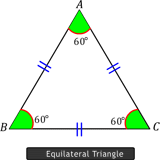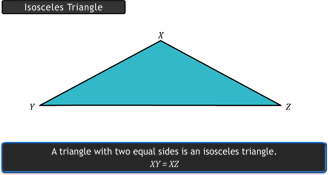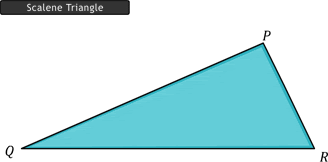Mark the mid-point of the side of a triangle, and join it to its opposite vertex. This line segment is called a median. It is defined as a line segment drawn from a vertex to the mid-point of the opposite side. You can draw three medians to a given triangle. The medians pass through a common point. Hence, the medians of a triangle are concurrent. This point of concurrence is called the centroid, and is denoted by G. The centroid and medians of a triangle always lie inside the triangle. The centroid of a triangle divides the median in the ratio 2:1.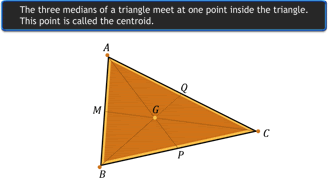Altitude: The altitude of a triangle is a line segment drawn from a vertex and is perpendicular to the opposite side. A triangle has three altitudes. The altitudes of a triangle are concurrent. The point of concurrence is called the orthocentre, and is denoted by O. The altitude and orthocentre of a triangle need not lie inside the triangle.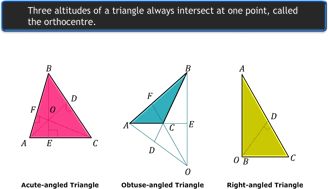Properties of Triangles

• An exterior angle of a triangle is equal to the sum of its interior opposite angles.
• The total measure of the three angles of a triangle is 180°.
• Sum of the length of any two sides of a triangle is greater than the length of the third side.
• In a right-angled triangle, the side opposite to the right angle is called the hypotenuse and the other two sides are called its legs.
• The Pythagoras Property states that in a right-angled triangle, the square of the hypotenuse is equal to the sum of the squares on the legs.
• If the Pythagoras Property holds, the triangle must be right-angled.

 With our free PDF notes you can get success in any competitive or entrance exams like CTET,  KVS, NET, CAT, MAT, CMAT, SSC, B.ED, IBPS Recruitment, IAS, CSAT, State Civil Services Exams, UPTET, PSTET, HTET & many more. It also provides NCERT solutions, CBSE, NTSE, Olympiad study material, Indian General Knowledge, English, Hindi, Mathematics, Current affairs, Science, S.ST, model test papers, important Questions and Answers asked in CBSE examinations.